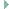arduino_board.h File Reference

Configuration of the Arduino API for Arduino Atmega boards. More...

## Detailed Description

Configuration of the Arduino API for Arduino Atmega boards.

Definition in file arduino_board.h.

`#include "arduino_pinmap.h"`
`#include "periph/pwm.h"`Include dependency graph for arduino_board.h:

Go to the source code of this file.

 #define ARDUINO_LED   (13) The on-board LED is connected to pin 13 on this board. #define ARDUINO_PWM_FREQU   (490U) PWM frequency. #define F_CPU   CLOCK_CORECLOCK F_CPU defines the CPU frequency in Hz. More... static const gpio_t arduino_pinmap [] Look-up table for the Arduino's digital pins. static const adc_t arduino_analog_map [] Look-up table for the Arduino's analog pins. More... static const arduino_pwm_t arduino_pwm_list [] List of PWM GPIO mappings. More...

## ◆ F_CPU

 #define F_CPU   CLOCK_CORECLOCK

F_CPU defines the CPU frequency in Hz.

This is used in AVR's libc delay.h and setbaud.h

In RIOT delay() has a different implementation using ztimer, and F_CPU is already defined when using setbaud.h (see cpu/atmega_common/periph/uart.c)

However Arduino libraries and sketches may expect F_CPU to be defined and fail otherwise (for example the Arduino SDI-12 package expects this, for AVR cpus). For this reason we define F_CPU here, if not already defined.

Definition at line 186 of file arduino_board.h.

## ◆ arduino_analog_map

static
Initial value:
= {
ARDUINO_A0,
ARDUINO_A6,
}
#define ARDUINO_A1
#define ARDUINO_A2
#define ARDUINO_A3
#define ARDUINO_A4
#define ARDUINO_A7
#define ARDUINO_A5

Look-up table for the Arduino's analog pins.

Definition at line 124 of file arduino_board.h.

## ◆ arduino_pwm_list

 const arduino_pwm_t arduino_pwm_list[]
static
Initial value:
= {
{ .pin = 6, .dev = PWM_DEV(0), .chan = 0 },
{ .pin = 5, .dev = PWM_DEV(0), .chan = 1 },
{ .pin = 11, .dev = PWM_DEV(1), .chan = 0 },
{ .pin = 3, .dev = PWM_DEV(1), .chan = 1 },
}
#define PWM_DEV(x)
Default PWM access macro.
Definition: pwm.h:78

List of PWM GPIO mappings.

Definition at line 153 of file arduino_board.h.